• 1.编程求解1!+2!+3!+…+19!+20!的值。 代码: #include <iostream> using namespace std; int main(){ int sum = 0; int n = 1; for(int i = 1;...2.已知四位数a2b3能被23整除，编程求出
1.编程求解1!+2!+3!+…+19!+20!的值。

代码:

#include <iostream>
using namespace std;

int main(){
int sum = 0;
int n = 1;
for(int i = 1;i <= 20;i++){
n = n * i;
sum = sum + n;
}
printf("阶乘结果：%d",sum);
return 0;
}


运行结果: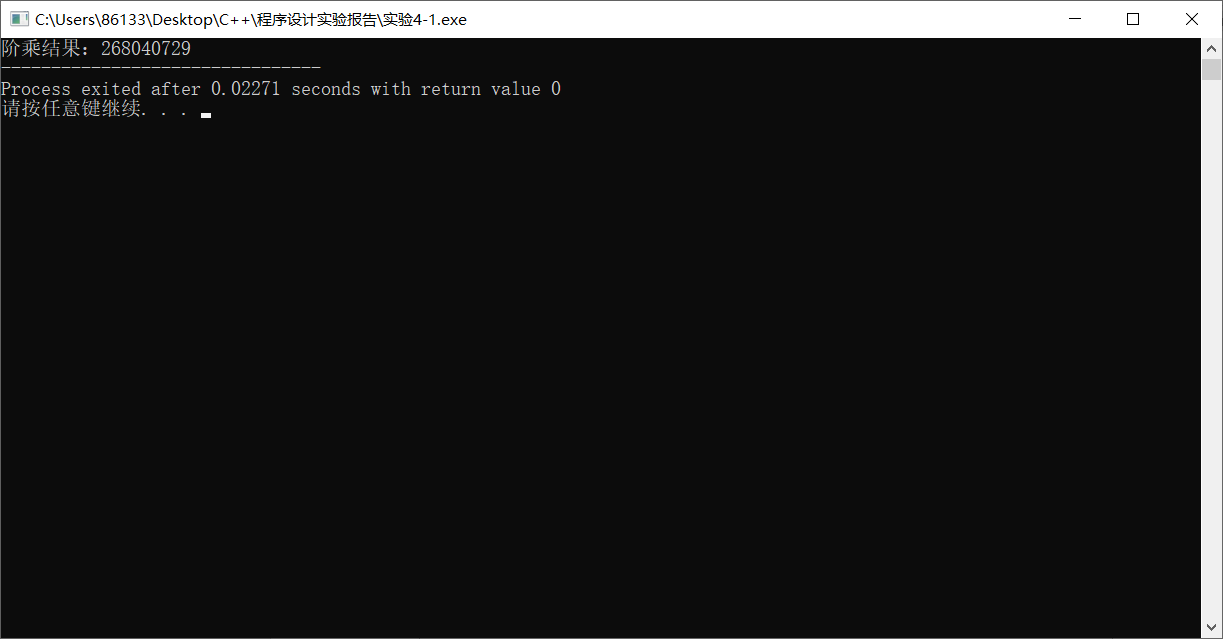2.已知四位数a2b3能被23整除，编程求出此数。

代码：

#include <iostream>
using namespace std;

int main(){
for(int i = 1000;i < 10000;i++){
if(i%23==0 && i/100%10==2 && i%10==3)
printf("%d\n",i);
}
return 0;
}


运行结果：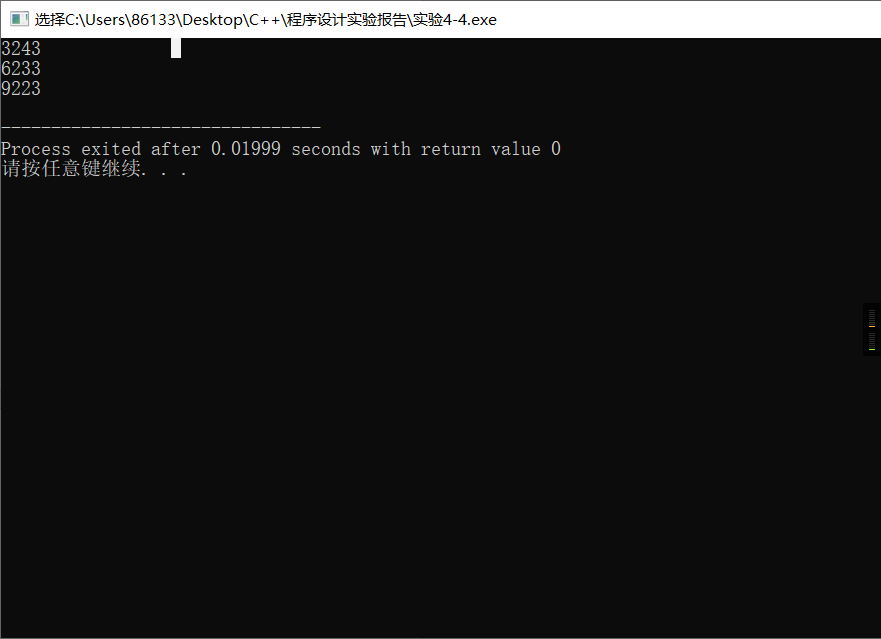3.输入一行字符，分别统计出其中英文字母、空格、数字和其它字符的个数。

代码：

#include <iostream>
using namespace std;

int main(){
char str;
printf("输入字符串；");
gets(str);
int sum1 = 0,sum2 = 0,sum3 = 0,sum4 = 0;
for(int i = 0;str[i] != '\0';i++){
if((str[i]>='A'&&str[i]<='Z') || (str[i]>='a'&&str[i]<='z'))
sum1++;
else if(str[i] == ' ')
sum2++;
else if(str[i] >= '0' && str[i] <= '9')
sum3++;
else
sum4++;
}
printf("英文字母的个数:%d\n",sum1);
printf("空格的个数:%d\n",sum2);
printf("数字的个数:%d\n",sum3);
printf("其它字符的个数:%d",sum4);
return 0;
}


运行结果: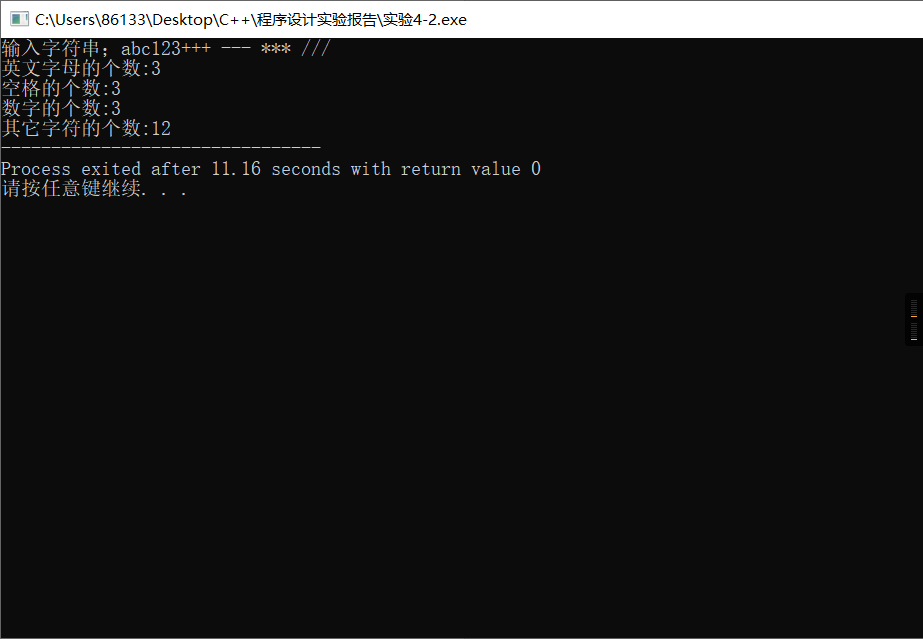4.编程打印以下图案。

********

******

****

**

****

******

********

代码:

#include <iostream>
using namespace std;

int main(){
for(int i = 1;i <= 4;i++){
for(int j = 1;j <= 8;j++){
if((j<=9-i) && (j>=i))
putchar('*');
else
putchar(32);
}
putchar('\n');
}
for(int i = 4;i <= 7;i++){
for(int j = 1;j <= 8;j++){
if((j<=i+1) && (j>=8-i))
putchar('*');
else
putchar(32);
}
putchar('\n');
}
return 0;
}


运行结果: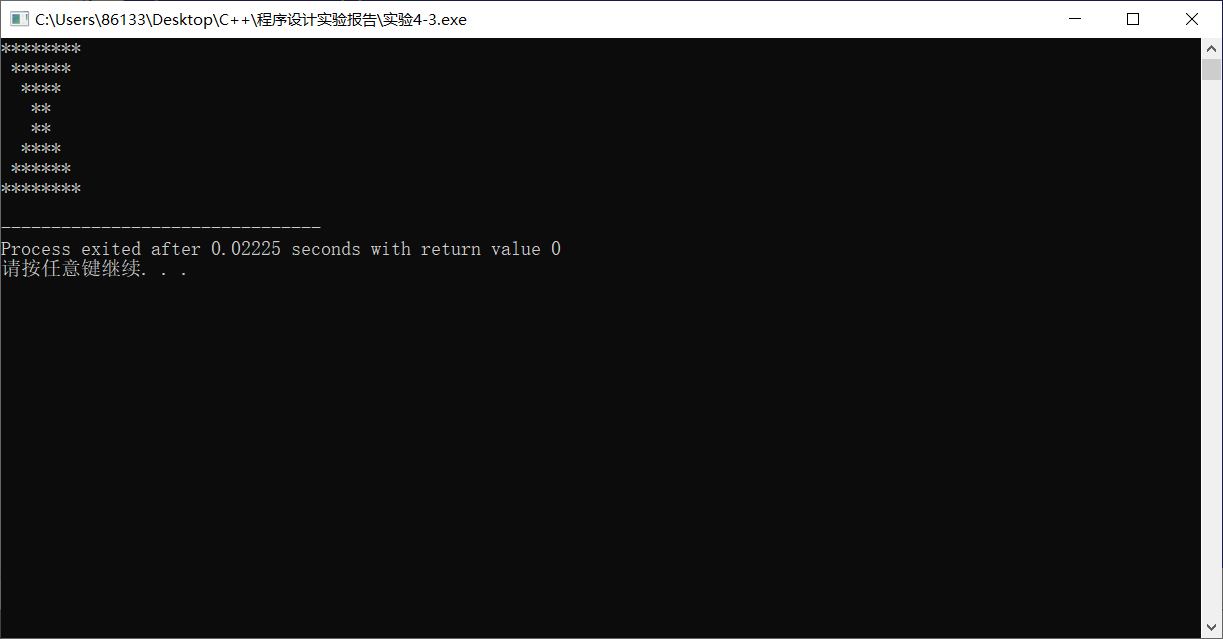展开全文c++
• 由于for语句在c语言中特别重要，所以今天主要学习内容为：复习for语句相关知识、练习习题（例题） 不练不知道，一练吓一跳！！！ 真的看懂和自己会，是两码事！ 自己会&敲对也是两码事！所以学习编程语言一定要...

由于for语句在c语言中特别重要，所以今天主要学习内容为：复习for语句相关知识、练习习题（例题） 不练不知道，一练吓一跳！！！
真的看懂和自己会，是两码事！ 自己会&敲对也是两码事！所以学习编程语言一定要自己敲代码、自己思考，真正做到自己背着书敲对才是学会——掌握。

话不多说，开始例题：
1-1：
用for语句编写程序求1+2+3+…+100的和。
#include <stdio.h>
int main ()
{
int i,sum=0;
for(i=1;i<=100;i++)
sum=sum+i;
printf("sum=%d\n",sum);
}

1-2:
编程输入1——200之间的所有用3除余2，且用5除余3的数，并统计这样的数有多少个。
#include <stdio.h>
int main ()
{
int i,sum=0;
for(i=1;i<=200;i++)
if((i%3==2)&&(i%5==3))
{
printf("%4d",i);
sum++;
}
printf("\n这样的数有：%d个",sum);
}

1-3
重新练习了一下鸡兔同笼的问题，还是暴露 了问题。
由于时间原因，今天笔记就整理这么多，谢谢你看到这里~ see u tomorrow！ 晚安！


展开全文• C语言提供for、while、do-while三种循环语句来实现循环结构。 1.while语句属于当型循环，用于循环次数未知，条件控制的循环。while循环的一般形式为： while（表达式） ｛ 循环体语句 ｝ 2.for语句用于实现当型循环...
循环语句是在C语言经常遇到的一种控制语句。在实际应用中的许多问题，都会涉及重复执行一些操作，如级数求和、穷举或迭代求解等。
C语言提供for、while、do-while三种循环语句来实现循环结构。
1.while语句属于当型循环，用于循环次数未知，条件控制的循环。while循环的一般形式为：
while（表达式）
｛
循环体语句
｝
2.for语句用于实现当型循环控制结构。在循环顶部进行循环条件测试，如果循环条件第一次测试为假，则循环体一次也不执行。for循环的一般形式为：
for（表达式1；表达式2；表达式3）
｛
循环体语句
｝
3.do-while语句用于实现直到型循环控制结构。在循环底部进行循环条件测试，循环至少执行一次。do-while循环的一般形式为：
do｛
循环体语句
｝while（表达式）；
另外再本章学习中还学习到了“流程的转移控制”学习了三个语句，分别是goto语句；break语句；continue语句。这三种语句中break语句使用的比较多。举一个例子来看一下：
韩信有一队兵，他想知道有多少人，便让士兵排队报数。按从1至5报数，最末一个士兵报的数为1；按从1至6报数，最末一个士兵报的数为5；按从1至7报数，最末一个士兵报的数为4；最后再按从1至11报数，最末一个士兵报的数为10。你知道韩信至少有多少兵吗？
1.使用基本的for循环（穷举法）
#include <stdio.h>
int main()
{
int  x;
for (x=1; x < 5000 ;x++)
{
if (x%51 && x%65 && x%74 && x%1110)
{
printf(“x = %d\n”, x); }
}
}
2.使用break语句
int main()
{
int  x;
for (x=1;  ;x++)
{
if (x%51 && x%65 && x%74 && x%1110)
{
printf(“x = %d\n”, x);
break;
}
}
}
我个人觉得在实际做题练习中，单独用到一个循环语句的时候并不多，大多数都是使用多个循环语句，即循环嵌套语句。所谓的循环嵌套语句通俗意义上讲就是循环里面套循环。
现在来句几个比较简单的嵌套循环的例子：
1.打印九九乘法口诀表
#include
int main()
{
int i = 0;
int j = 0;
for (i = 1; i <= 9; i++)
{
for (j = 1; j <= i; j++)
{
printf("%d*%d=%d “, i, j, i*j);
if (i == j)
printf(”\n");
}
}
return 0;
}
2.输入一个数判断是否为素数。素数是只能被一和自身整除的数。
#include
#include
using namespace std;
int main()
{
int i,j,k;
bool prime;
char c=‘Y’;
while(c==‘Y’)
{
cout<<“请输入一个整数：”;
cin>>i;
k=int(sqrt(i));
prime=true;
for(j=2;j<=k;j++)
{
if(i%j==0)
{
prime=false;
cout<<“不是”<<endl;
break;
}
}
if(prime)
{
cout<<“是”<<endl;
}
cout<<“是否继续判断，输入Y继续，输入其他字符，退出程序:”;
cin>>c;
}
cout<<“退出程序！”<<endl;
return 0;
}
这几个例子就充分体现了循环嵌套的应用。
总结：
我认为循环语句是学习c语言的一个重要基础，因为后面在学习其他复杂的结构时，几乎都会涉及到循环语句。所以以后还要继续加强训练。


展开全文• 第五章 循环结构程序设计例5.1 求1+2+3+…+100.例5.2 用do…while求1+2+3+…+100.例5.3 while与do while比较例 5.4 在全系1000名学生中举行慈善捐募，当总数达到10万元时就结束，统计此时捐款人数以及平均没人捐款...
第五章 循环结构程序设计例5.1 求1+2+3+…+100.例5.2 用do…while求1+2+3+…+100.例5.3 while与do while比较例 5.4 在全系1000名学生中举行慈善捐募，当总数达到10万元时就结束，统计此时捐款人数以及平均没人捐款数目。例5.5 要求输出100~200的不能被3整除的数。例5.6 输出4*5矩阵例5.7 用公式π/4≈1-1/3+1/5-1/7+…求π的近似值直到发现某一项的绝对值小于10的-6次方为止例5.8 求斐波那契数列的前40个数例5.9 输入一个大于3的整数n，判定它是否为素数。例5.10求100~200的全部素数例5.11译密码
例5.1 求1+2+3+…+100.
int main()
{
int i=1,x=0;
while (i<=100)
{
x=x+i;
i++;
}
printf("x=%d",x);
return 0;
}

运行结果如下：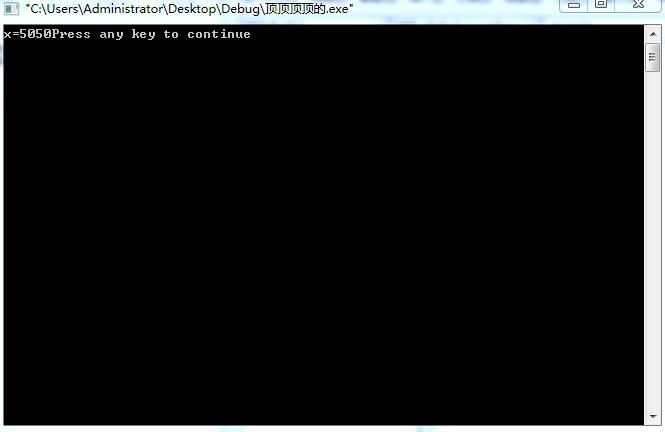例5.2 用do…while求1+2+3+…+100.
int main()
{
int i=1,x=0;
do
{
x=x+i;
i++;
}while(i<=100);
printf("x=%d",x);
return 0;
}

运行结果如下：例5.3 while与do while比较
while
int main()
{
int i,sum=0;
printf("please enter i,i=?");
scanf("%d",&i);
while(i<=10)
{
sum=sum+i;
i++;
}
printf("sum=%d\n",sum);
return 0;
}

运行结果如下：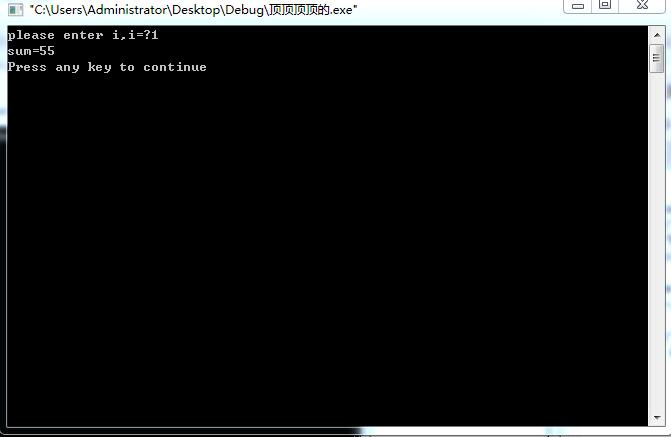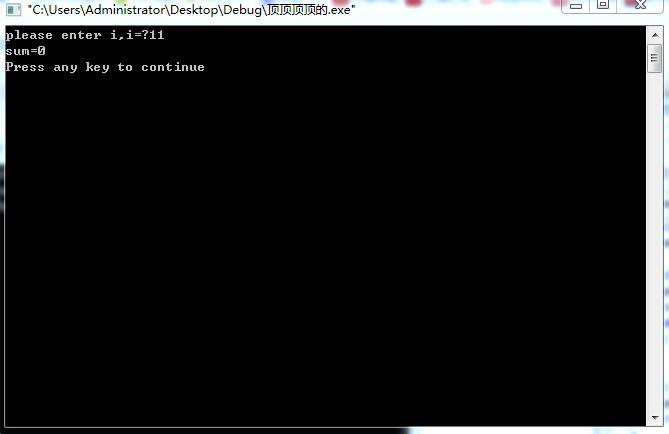do whlie
# include <stdio.h>
int main()
{
int i,sum=0;
printf("please enter i,i=?");
scanf("%d",&i);
do
{
sum=sum+i;
i++;
}while(i<=10);
printf("sum=%d\n",sum);
return 0;
}

运行结果如下：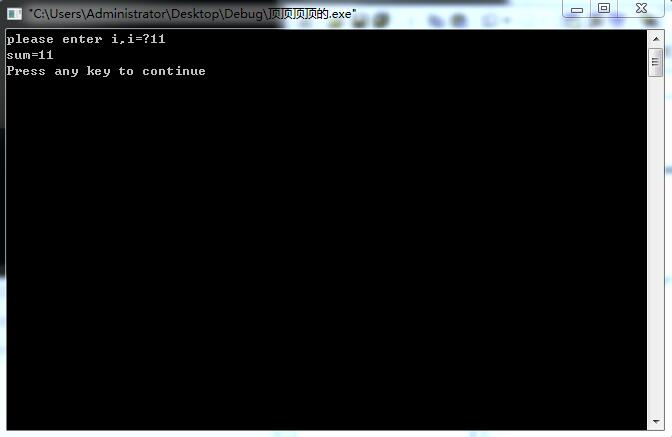例 5.4 在全系1000名学生中举行慈善捐募，当总数达到10万元时就结束，统计此时捐款人数以及平均没人捐款数目。
# include <stdio.h>
int main()
{
float amount,aver,total=0;
int i;
for(i=1;i<=1000;i++)
{
printf("please enter amount:");
scanf("%f",&amount);
total=total+amount;
if(total>=10000)
break;
}
aver=total/i;
printf("num=%d\naver=%f\n",i,aver);
return 0;

}

运行结果如下：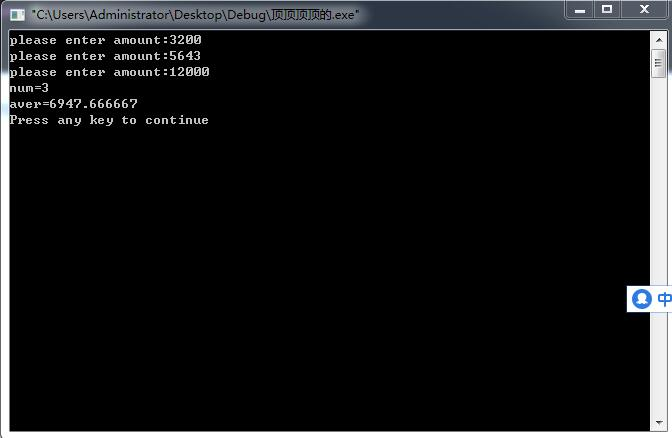例5.5 要求输出100~200的不能被3整除的数。
# include <stdio.h>
int main()
{
int x;
for(x=100;x<=200;x++)
{	if(x%3!=0)
printf("%d\t",x);}
return 0;
}

运行结果如下：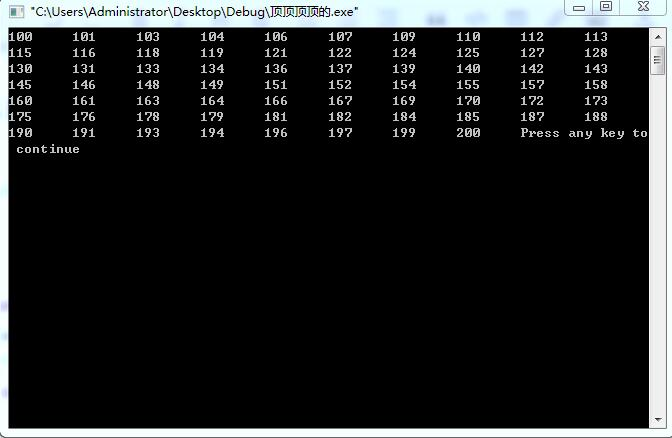例5.6 输出4*5矩阵
# include <stdio.h>
int main()
{
int x,j,n=1;
for(x=1;x<=4;x++)
for(j=1;j<=5;j++,n++)
{ 	printf("%d\t",x*j);
if(n%5==0)printf("\n");

}
return 0;
}

运行结果如下：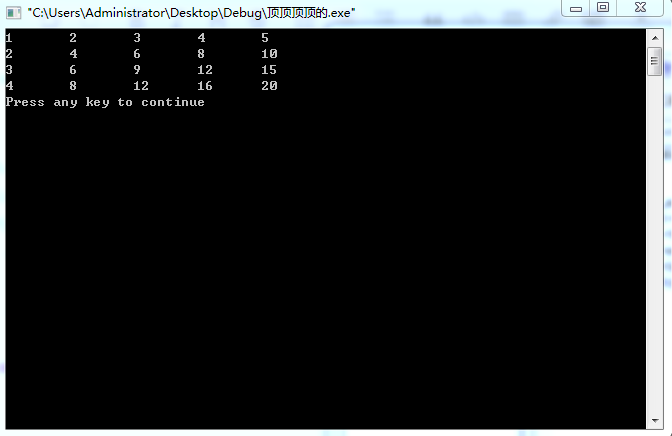# include <stdio.h>
int main()
{
int x,j,n=0;
for(x=1;x<=4;x++)
for(j=1;j<=5;j++,n++)
{ 	if(n%5==0)printf("\n");
if(x==3&&j==1)
break;

printf("%d\t",x*j);
}
return 0;
}

运行结果如下：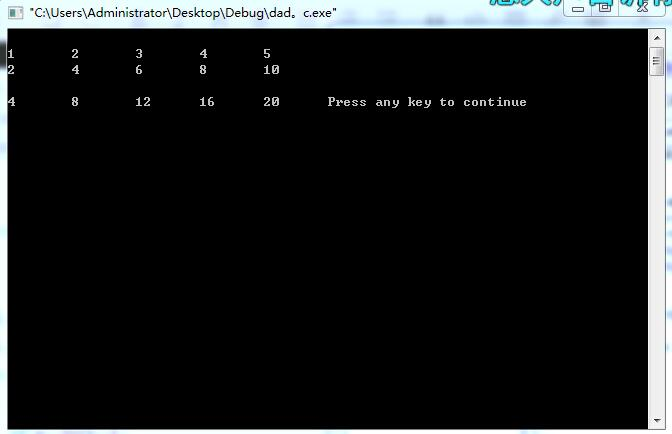# include <stdio.h>
int main()
{
int x,j,n=0;
for(x=1;x<=4;x++)
for(j=1;j<=5;j++,n++)
{ 	if(n%5==0)printf("\n");
if(x==3&&j==1)
continue;

printf("%d\t",x*j);
}
return 0;
}

运行结果如下：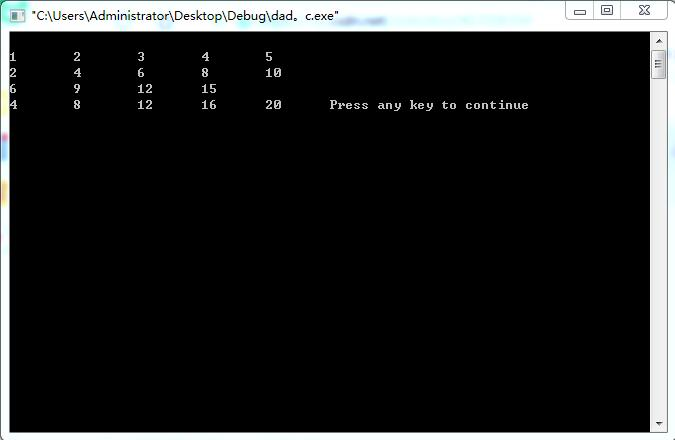例5.7 用公式π/4≈1-1/3+1/5-1/7+…求π的近似值直到发现某一项的绝对值小于10的-6次方为止
# include <stdio.h>
# include <math.h>
int main()
{
int sign=1;
double n=1.0,sum=0.0,term=1.0;
while(fabs(term)>=1e-6)
{	sum=sum+term;
sign=-sign;
n=n+2;
term=sign/n;}
sum=sum*4;
printf("sum=%10.8f\n",sum);
return 0;
}

运行结果如下：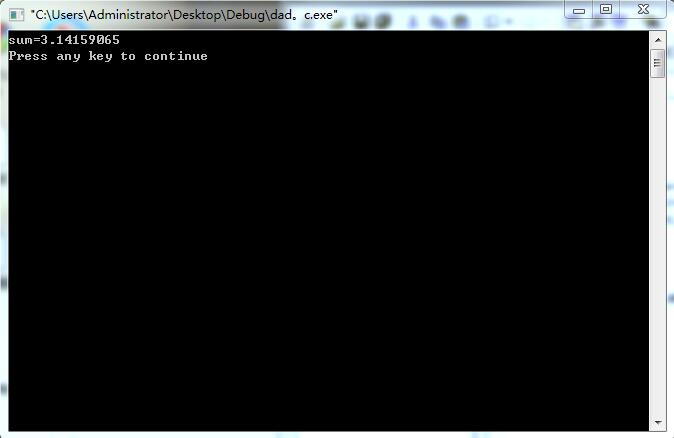例5.8 求斐波那契数列的前40个数
# include <stdio.h>
int main()
{
int x=1,y=1,z;
int i;
printf("%12d\n%12d\n",x,y);
for(i=1;i<=38;i++)
{	z=x+y;
x=y;
y=z;
printf("%12d\n",z);
}
return 0;
}

运行结果如下：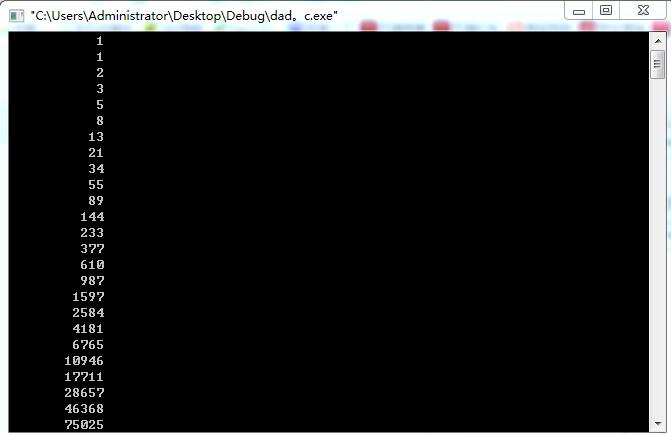# include <stdio.h>
int main()
{
int x=1,y=1,z;
int i;
for(i=1;i<=20;i++)
{	printf("%12d%12d",x,y);
if(i%2==0)printf("\n");
x=y+x;
y=y+x;
}
return 0;
}

运行结果如下：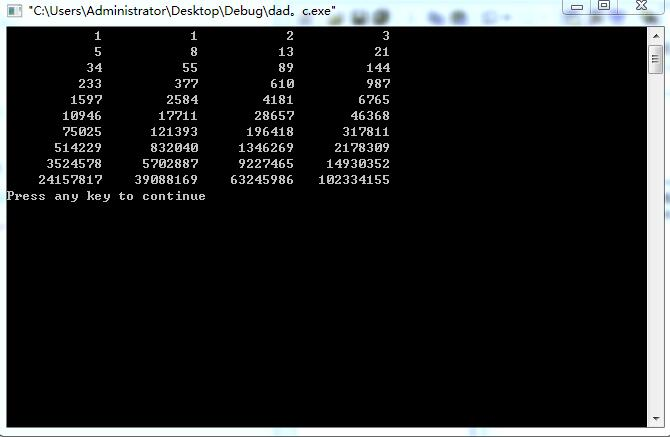例5.9 输入一个大于3的整数n，判定它是否为素数。
# include <stdio.h>
int main()
{
int n,i;
printf("please enter a integer number,n=?");
scanf("%d",&n);
for(i=2;i<n;i++)
{
if(n%i==0)
break;
}
if(i<n)
printf("该数不为素数");
if(i=n)
printf("该数为素数");
return 0;
}

运行结果为：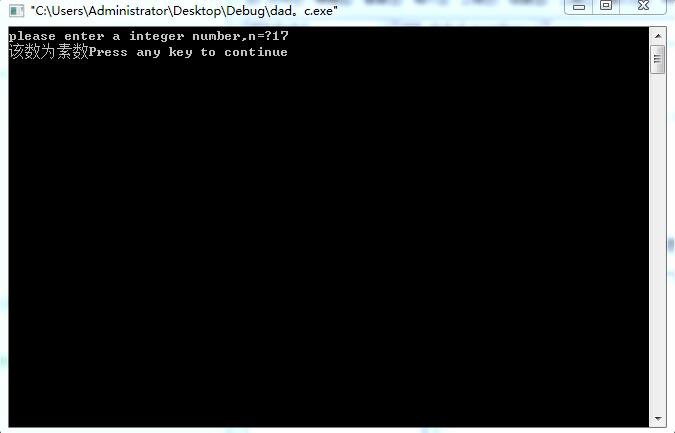# include <stdio.h>
# include <math.h>
int main()
{
int n,i,k;
printf("please enter a integer number,n=?");
scanf("%d",&n);
k=sqrt(n);
for(i=2;i<=k;i++)
{
if(n%i==0)
break;
}
if(i<k)
printf("该数不为素数");
else
printf("该数为素数");
return 0;
}

运行结果如下：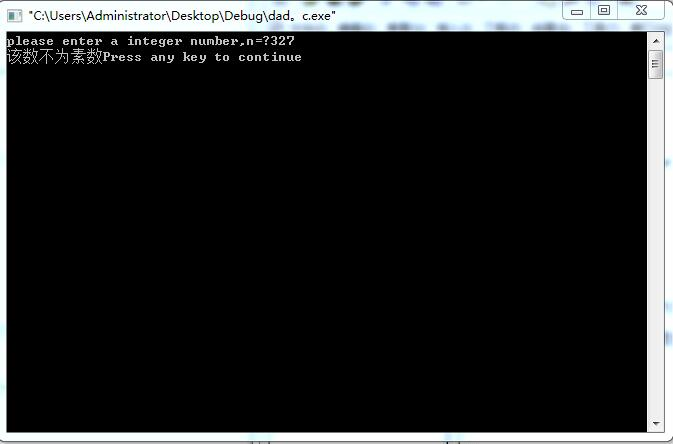例5.10求100~200的全部素数
# include <stdio.h>
# include <math.h>
int main()
{
int k,i,n,m=0;
for(n=100;n<=200;n++)
{
k=sqrt(n);
for(i=2;i<=k;i++)
{	if(n%i==0)
break;}
if(i>k)
{printf("%d ",n);
m=m+1;}
if(m%10==0)
printf("\n");
}
return 0;
}

运行结果如下：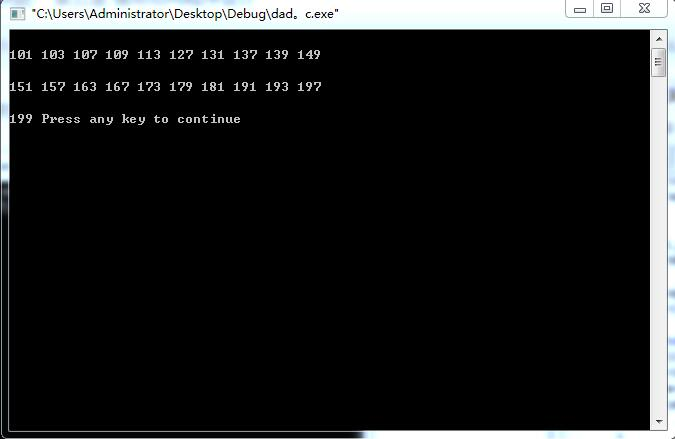例5.11译密码
# include <stdio.h>
int main()
{char c;
c=getchar();
while(c!='\n')
{	if(c>='a'&&c<='z'||c>='A'&&c<='Z')
{if(c>='w'&&c<='z'||c>='W'&&c<='Z')
c=c-22;
else
c=c+4;
}
printf("%c",c);
c=getchar();
}
printf("\n");
return 0;

}

运行结果如下：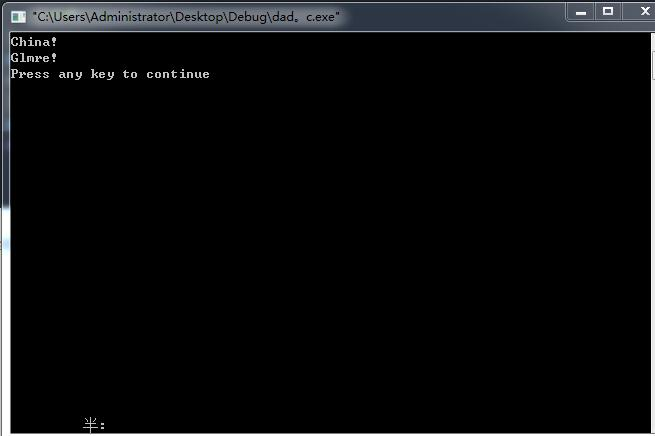展开全文• 有关于c语言的基础例题，仅供参考 1． 使用字符串处理函数，要包含string.h头文件 。使用输入输出函数要包含stdio.h。使用数学函数，要包含math.h头文件。 2． 字符串结束标志符是’\0’。”asdgggg” 3． x++是先...
• C语言程序设计（第3版）何钦铭 第四章循环结构 课本的例题以及练习
• C语言经典例题 小测五道题 小编想说：我是我们学校软件设计和程序协会研发部的一员，我们研发部每星期都会在我们的公众号上分享一些小题目。最近都是出C语言类的，这星期是我选题目。以下就是我这一初学者的拙作，...程序设计
• 例题： 代码如下： 判断单链表中是否有环？ 有环的定义是，链表的尾结点指向了链表中的某个结点。如： 判断单链表是否有环，主要有以下两种方法： 代码太长了，我又头大了 小甲鱼老师讲的真的好详细！！ ...链表
• 近期，C语言的第三套练习题目也已经结束，就循环结构也偶一定的心得体会在此交流。  循环结构给我的印象就是灵活性大，难度比前五章的学习中上了个档次，刚开始接触，一头雾水，丝毫没有头绪，接着参考书对比着...
• 循环控制结构程序之例题 几种循环的比较 （1） 四种循环都可以用来处理同一问题，一般情况下他们可以互相代替。但是一般不提倡用goto型循环。 （2） 在while循环和do-while 循环中，只在while后面的括号内指定循环...
• 全面解析C语言：C_入门，声明，表达式，C_数组与函数，C_选择结构循环结构
• 所有语言的结构总共有三种，分别是顺序结构、选择结构和循环结构C语言也不例外。 一、顺序结构 所谓顺序结构就是按照语句的书写顺序，一步一步运行。 //例题： #include <stdio.h> #include <stdlib....顺序结构 选择结构# c语言循环结构例题c语言 订阅